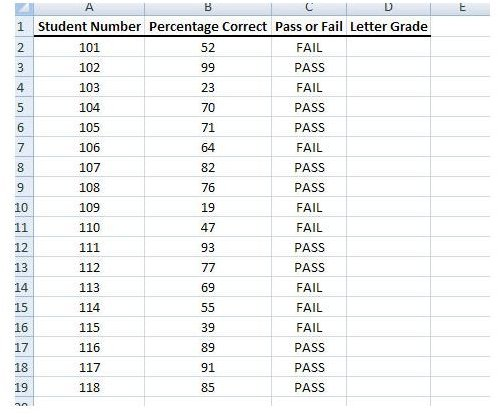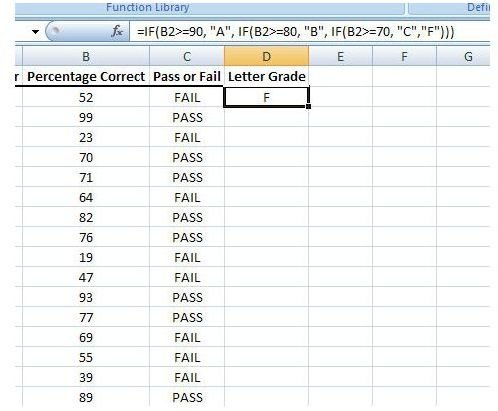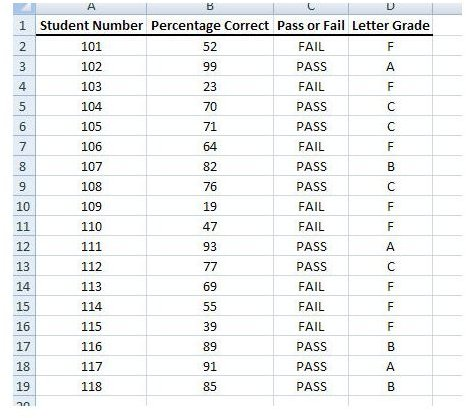# How to Use Nested IF Functions in Excel 2007 to Calculate Letter Grades

Page content

## Nested IF Functions

At first glance, the format of a basic IF function in Excel may lead some users to believe that the function can only be employed in times when there are just two choices to be made such as in the example we gave in Part 1 of this series. However, it is possible to use IF statements when there are more than two cases if the functions are nested correctly.

A nested IF function is a statement that contains at least one IF function inside another. That is, a nested IF function contains another IF statement as one (or both) of the true/false values. In this manner, the original IF function checks for a condition and, depending on the result of that check, it may go on to check another condition. The diagram to the left gives a basic representation of how this process works. (Click the image for a larger view.)

A basic IF function is of the form below.

=IF(condition, value if true, value if false)

A more concrete example that we created in Part 1 of this series is the function IF(B2>=70,“PASS”,“FAIL”).

On the other hand, nested IF functions can take on a variety of different appearances, depending on how complex the nesting sequence is. One example is shown below.

=IF(condition1, value1 if true, IF(condition2, value2 if true, value2 if false))

## Extending the Example

Let’s take a look at the example we gave in Part 1 again. Suppose now, that instead of wanting to just assign a grade of Pass or Fail, that the instructor wants to assign the letter grades A, B, C, or F based on the following rules.

• If the percentage correct is 90 or higher, the student will receive an A.
• If the percentage is 80 or higher but less than 90, the student will receive a B.
• If the percentage is 70 or higher but less than 80, the student will receive a C.
• All other students will receive an F.

We’ll add a new column to the spreadsheet used in Part 1 of this series to account for the letter grade.Since we’re using a nested IF function, we need to approach this a little different than we did in Part 1. Instead of selecting the function tool from the Formula tab, we’ll just select the first empty cell in the Letter Grade column and type in the following formula.

=IF(B2>=90, “A”, IF(B2>=80, “B”, IF(B2>=70, “C”,“F”)))Then, we’ll copy the formula and paste it into the other cells of the column.## Limitations of Nested IF Functions

While previous versions of Excel limited users to no more than seven levels of nested IF functions, Excel 2007 supports up to 64 nested functions. Does that mean you want to use an IF function for situations when you have dozens of conditions? You could, but I wouldn’t recommend it. There are better, more efficient functions to use in situations like this. We’ll be covering them later in this series.

## This post is part of the series: How to Use Logical Functions in Microsoft Excel 2007

The logical functions of Microsoft Excel 2007 are extremely powerful tools that be used for many applications. This group of articles takes a look at individual logical functions available in Excel and describes how they can be used to save you time and improve your project analysis.